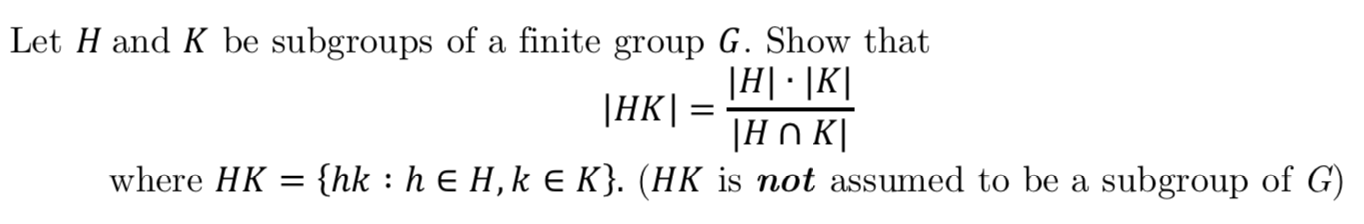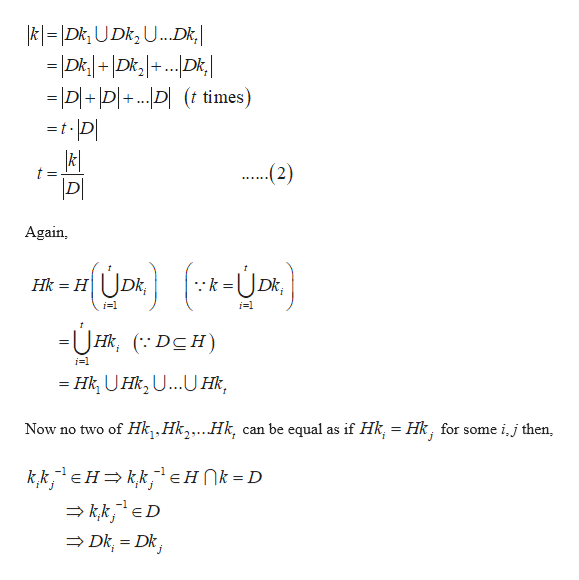# Let H and K be subgroups of a finite group G. Show that|HK|HK=|HОКIwhere HK (hk hE H, k E K}. (HK is not assumed to be a subgroup of G):

Questionhelp_outlineImage TranscriptioncloseLet H and K be subgroups of a finite group G. Show that |HK |HK= |HОКI where HK (hk hE H, k E K}. (HK is not assumed to be a subgroup of G) : fullscreen
check_circleExpert Solution
Step 1

let D = HK then D is a subgroup of k and there exist a decomposition of k into disjoint right cosets of D in k and

Step 2

This impl...help_outlineImage Transcriptionclose|k=|Dk; U Dk,U..Dk, = Dk+|Dk+..D ID+|D|+..D (t times) ..(2) t = Again Hk H Dk; i1 i1 =UHk, DCH) i1 =Hk UHk,U...U Hk Now no two of Hk,,Hk,.. .Hk, can be equal as if Hk, = Hk, for some i,j then, kkHkkEHNk = D kk,ED Dk, Dk, fullscreen

### Want to see the full answer?

See Solution

#### Want to see this answer and more?

Solutions are written by subject experts who are available 24/7. Questions are typically answered within 1 hour*

See Solution
*Response times may vary by subject and question
Tagged in

### Math